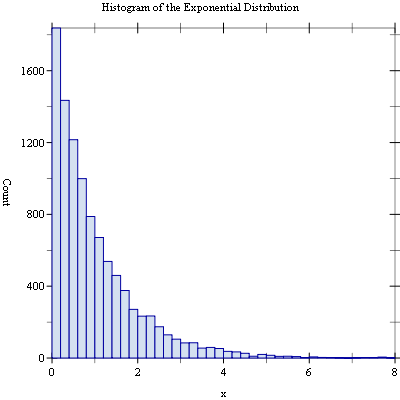Question: Transformation Of A Quantitative Trait In Gwas
0
kakukeshi50 wrote:

Hi there,

I want to perform GWAS on different quantitative traits that follow different distributions.

Some traits follow a near-normal distribution so I can normalize them by using Inverse Normal Transformation (by the way is this necessary?). While other traits follow an exponential distribution with many individuals (y-axis) with a value of 0 (x-axis) as is shown in the image below. Do I need to transform this distribution to normal and if so, how can I do it?

Many thanks guysgwas • 514 views